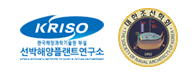Instructions to participants
Case 3.3: KCS Waves, trajectories

# CASE 3.3: KCS in deep water in waves

## 1. Description of case 3.3

• KCS hull shape, at even keel draught of 10.8 meter (full scale), model scale draught 0.1435m.
• LPP = 3.057 m (scale 75.24)
• No bilge keels.
• Free to move in all directions.
• Starting speed: Fn = 0.16 (corresponding to full scale 14.5 knots).
• Calm water
• g = 9.81 [m/s2], ρ=1000 [kg/m3]; ν=1.27×10-6 [m2/s]
• Propeller present and working at constant RPM. The RPM of the propeller is such that the ship is in (longitudinal) equilibrium at a speed of 14.5 knots full scale.
• Rudder (mariner type) steerable. The rudder rate will correspond to 2.32°/s on full scale or 20.1°/s on model scale
• GM is set to 0.6 meter (full scale), model scale value of 0.008 m)
• Water depth is deep water.
• Radius of gyration for yaw and pitch is 0.25LPP (in air)
• Radius of gyration for roll is 0.49B (in water)

For the test in waves:
• Regular waves
• Wave height 48 mm on model scale, (3.61 m on full scale)
• Wave length to ship length ratio = 1.0, meaning that the wave frequency is 4.49 rad/s on model scale (0.518 rad/s on full scale). Consequently, the wave period is 1.4 s on model scale (12.14 s on full scale)
• Wave direction: head waves with the ship approaching t=0
• The rudder execute (t=0) is when a wave bottom is passing midship.
Case 3.3 consists of 2 cases: case 3.3.1 through 3.3.2, as explained below:

## 2. Experimental data

The submissions will be compared to model tests carried out by Hiroshima University. Furthermore, there is 1 other set of measurements, of IIHR. They will help to obtain insight in the measurement uncertainty.

## 3. Requested computations

The requested info comes in 2 pieces. You can only deliver case 3.3.2 when you deliver case 3.3.1.

##### Table1: Requested information
Package Manoeuvre Starting speed V0
Case 3.3.1 35° turning circle test, starting to starboard side 0.86 m/s (equivalent to 14.5 knots full scale)
Case 3.3.2 35° turning circle test in waves, starting to starboard side 0.86 m/s (equivalent to 14.5 knots full scale)
The propeller RPM at t=0 should be such that the ship is in longitudinal equilibrium. So, for a CFD time domain simulations, the situation at t=0 is equivalent to a propulsion test! The analysers will look at this data.

## 3.1. Submission 3.3.1: Turning circle test in calm water at 14.5 knots

• All results are to be given for model scale conditions.
• All simulations results should be provided in the format described in section 4.
• The propeller rate of revolution should correspond to the self-propulsion point of the model. When the RPM is unknown (because for example a constant thrust or actuator disk model is used in CFD based simulations) please indicate this.
• Similar to the experiment, the rudders are controlled by autopilot during approaching before starting rudder execution.
• During the manoeuvre, the rudder goes to the real geometric -35 degrees (not to -35 degrees away from the neutral angle).
Please see section 5 for an example of the submission.

## 3.2. Submission 3.3.2: Turning circle test in waves at 14.5 knots

• All results are to be given for model scale conditions.
• All simulations results should be provided in the format described in section 4.
• The propeller rate of revolution should correspond to the self-propulsion point of the model. When the RPM is unknown (because for example a constant thrust or actuator disk model is used in CFD based simulations) please indicate this.
• Similar to the experiment, the rudders are controlled by autopilot during approaching before starting rudder execution.
• Regular wave with wave height 48 mm on model scale.
• During the manoeuvre, the rudder goes to the real geometric 35 degrees (not to 35 degrees away from the neutral angle).
Please see section 7 for an example of the submission.

## 4. Format

Time series of the manoeuvres should be send to the organizers in an excel file. The data shall be give on model scale, and it will be compared to model scale data.
The submission consists of an excel file (example is given) with 18 columns and with rows for every time step. The time step is free to chose, but a small enough time step somewhere around 0.02 to 0.01 is recommended to get sufficient accuracy. The following quantities are asked:

##### Table 2: Submission variables
Symbol Item Unit
t time s
x x position in earth fixed system m
y y position in earth fixed system m
z z position in earth fixed system m
ϕ Roll angle degrees
θ Pitch angle degrees
ψ Yaw angle degree
u surge velocity in horizontal-body-fixed coordinate system m/s
v sway velocity in horizontal-body-fixed coordinate system m/s
r yaw rate in body-fixed coordinate system deg/s
δ Rudder angle deg
n Propeller revolutions rpm
T Thrust of propeller N
Q Torque of propeller Nm
FR Rudder normal force (except horn part) N
These data are illustrated in Figure 1. The origin is located on O which is located at midships: the intersection of ordinate 10, the centre line plane and the still water waterline. The time t=0 is the time when the steering starts. The initial direction of the model is the direction of x0-axis. Initial conditions at t = 0 are: X0=0, Y0=0, u=U0, r=0, δ=δ0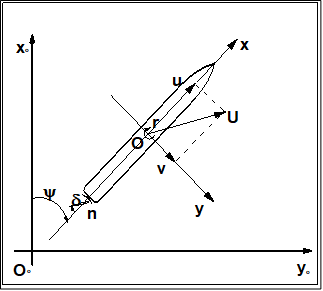Figure 1: Illustration of the sign convention of the earth-fixed and body-fixed coordinate system.

Explanation of the sign convention

Notes:
• The ship position or trajectory should be given in an Earth-fixed coordinate system with X pointing North, Y pointing East, and Z pointing downward as shown in Figure 1. This x0y0z0 axis system remains horizontal in the earth. The ship was released at (0,0) when t = 0 . The roll angle (ϕ) is positive for pushing starboard into the water, pitch (θ) is positive for bow up position and yaw angle (ψ) is positive for bow turned to starboard. The reported trajectory is dimensional and angular motions should be reported in degrees. The angular velocities should be reported in degree per second. The reported yaw angle should be the deviation of yaw angle respect to the target yaw i.e. ψ-ψC.
• All velocities for 6DOF motions (u, v, w p, q r) should be reported in ship-fixed coordinate system with x axis positive toward bow, y axis positive toward starboard and z axis positive downward. This xyz axis system rotates with the ship in yaw but not pitch and roll, i.e., remains horizontal in the earth. It is a so-called “yawed-only reference system”. Given this convention, the reported velocities are: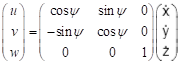(3)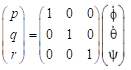(4)
In Equation (4), the unit of p, r,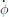, and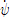is [deg/s]. Rudder angle (δ) is positive when trailing edge moves to portside.
• All motions should be reported at O.
There is a right-handed earth-fixed coordinate system x0y0z0 , and a horizontally moving right handed body-fixed coordinate system xyz. The body-fixed coordinate system has its origin in O, and the xy plane rotates with ship. The heading angle is defined as the angle between x0- and x-axis. u and v are the velocity components in x and y direction. the hull drift angle will be defined as β=atan(-v/u), and the total velocity U=√(u2+v2).

The ship position or trajectory should be given in an Earth-fixed coordinate system with x0 pointing North, y0 pointing East, and z0 pointing downward as shown in Figure 1. This axis system remains horizontal in the earth. The ship was released at (0, 0) when t = 0 . The roll angle (ϕ) is positive for pushing starboard into the water, pitch (θ) is positive for bow up position and yaw angle (ψ) is positive for bow turned to starboard. The reported trajectory is dimensional and angular motions should be reported in degrees. The angular velocities should be reported in degree per second. The reported starting yaw angle is zero. Rudder angle (δ) is positive when trailing edge moves to portside. The thrust T and torque Q for each propeller should be reported in shaft coordinate system with x axis positive toward the engine. Values are given dimensional

Time series of the manoeuvres should be send to the organizers in an excel file. The data shall be give on model scale, and it will be compared to model scale data.
The yellow fields in the excel sheet should be filled in. The excel sheet should be send to the organizing committee per email address “simman2019host@gmail.com”.

## 5. Elaborated example of case 3.3.1 (35° turning circle test, starting to starboard side)

For case 3.3.1, the comparison will look as displayed in the following figure 2. As can be seen, the results of the time traces will be analysed and presented non-dimensional. On top of the time traces as presented in figure 2, the values of tactical diameter, rate of turn, drift angle, speed loss, etc will be derived from the time series. Table 2 illustrated which characteristics will be derived from the time series.

The primary values for the comparison will be the advance and tactical diameter. The secondary values for the comparison are indicated in the table 2.

##### Table 2: Comparison values for Case 3.3.1
Category Unit Validation Variables
[-] Tactical diameter / LPP (AD/LPP)
Times [s] Time to reach 90° (t90°)
[s] Time to reach 180° (t180°)
[s] Time to reach 360° (t360°)
Yaw rate [deg/s] Maximum yaw rate (rmax)
[deg/s] Average yaw rate in the constant part of the turn (rC)
Speed [m/s] Speed in the steady part of the turn (UC)
Roll [deg] Maximum inward roll angle (ϕinmax)
[deg] Maximum outward roll angle (ϕoutmax)
[deg] Roll angle in the constant part of the turn (ϕC)
Non-dimensional values [-] Non-dimensional yaw rate in the constant part of the turn (r’)
[deg] Drift angle in the constant part of the turn (βC)
[-] Pivot point (-sin(βC)/r’)
[-] Speed ratio (UC/U0)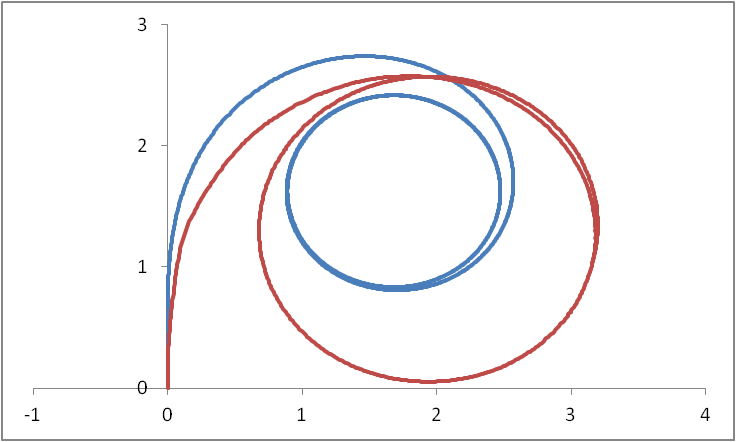Figure 2: time traces of a submission for the 35 degrees turning circle manoeuvre, compared to FRMT

## 6. Elaborated example of case 3.3.2 (35° turning circle manoeuvre, starting to startboard side IN WAVES)

For case 3.3.2, the comparison will look as displayed in the following figure 3. As can be seen, the results of the time traces will be analysed and presented non-dimensional. On top of the time traces as presented in figure 3, the values of tactical the average drift and the drift distance will be calculated. Table 3 illustrated which characteristics will be derived from the time series. These characteristics will be compared.

##### Table 3: Comparison values for Case 3.3.1 (see also figure 4)
Category Unit Validation Variables
Dimensions [-] Drift angle μD
[-] Drift distance HD / LPP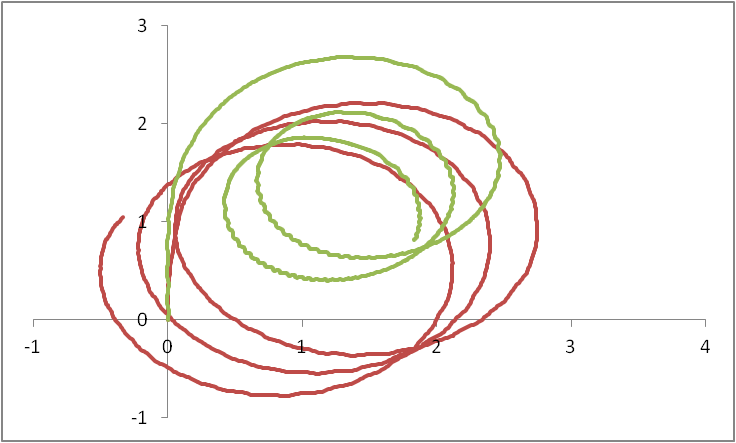Figure 3: time traces of a submission for the 35 degrees turning circle manoeuvre, compared to FRMT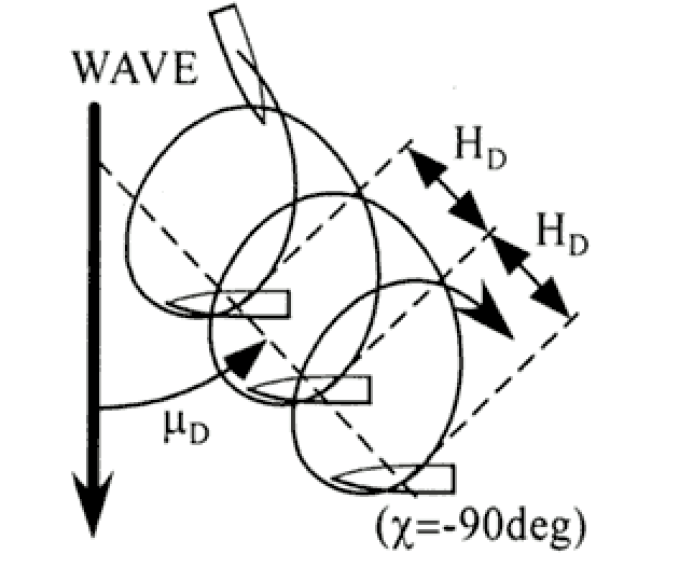Figure 4: Analysis of trajectory in waves
(34103) (171 Jang-dong) 32 1312beon-gil, Yuseong-daero, Yuseong-gu, Daejeon, KOREA